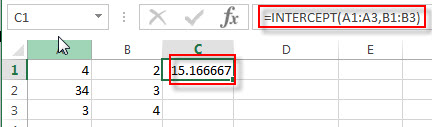# Excel INTERCEPT Function

This post will guide you how to use the INTERCEPT function with syntax and examples in Microsoft excel.

### Description

The Excel INTERCEPT function calculates the point at which a line will intersect the y-axis by using existing x-values and y-values. So it will return the y-axis intersection point of a line based on the existing x-axis values and y-axis values.

The INTERCEPT function is a build-in function in Microsoft Excel and it is categorized as a Statistical Function.

The INTERCEPT function is available in Excel 2016, Excel 2013, Excel 2010, Excel 2007, Excel 2003, Excel XP, Excel 2000, Excel 2011 for Mac.

### Syntax

The syntax of the INTERCEPT function is as below:

`=INTERCEPT (known_y's, known_x's)`

Where the INTERCEPT function arguments are:

• known_y’s – This is a required argument.  The known y-values or range of data used to calculate the intersection.
• known_x’s -This is a required argument.  The known x-values or range of data used to calculate the intersection.

Note:

• The arguments should be numbers, or arrays, or references that contains only numeric values.
• If any array or reference argument contains text, logical values, or empty cells, the INTERCEPT function will ignore those values.
• If known_y’s and known_x’s arrays have a different lengths, or do not contain any values, the INTERCEPT function will return the #N/A Error.

### Excel INTERCEPT Function Examples

The below examples will show you how to use Excel INTERCEPT Function to calculate the value at the intersection of the y-axis through a supplied set of x-values and y-values.

Example 1:   to calculate the point at which a line will intersect the y-axis by using the existing x-values A1:A3 and y-values B1:B3, using the following formula:

`=INTERCEPT(A1:A3,B1:B3)`